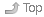# 1 Shell integer arithmeticIndexThe shell is not a normal programming language and it does not do arithmetic or mathematics well. In particular, the shell only accepts integer numbers – no floating-point (real, decimal) numbers.

Even integer arithmetic was not built-in to the original shells. To do arithmetic, the shells once used “helper” commands. Modern shells now have the arithmetic built-in to the shell, but many, many older shell scripts still use the old helper commands.

There are several historic ways to use helper commands to allow the shell to do integer arithmetic.

Shells don’t do math on floating-point (real) numbers (numbers with decimal points, e.g. `3.7`). If you want to do floating-point arithmetic, you really do need to use a helper program such as `dc` or `bc`.

## 1.1 The universal `expr` arithmetic helper commandIndexThe oldest and most universal arithmetic helper command is the `expr` command, and it is often used in older scripts inside the historic back-quote form of Command Substitution to capture its output value:

``````\$ expr 2 + 2 \* 9
20

\$ x=`expr 2 + 2 \* 9`                 # historic `...` command substitution syntax
\$ echo "\$x"
20``````

The individual arguments to the `expr` command constitute the arithmetic expression to be evaluated and the value is printed on standard output. Shell meta-characters such as `*` (GLOB) must be quoted to hide them from the shell. All numbers and operators must be individual arguments, separated by blanks, otherwise `expr` simply echoes the unrecognized expression back to the user without any error message and without doing any mathematics:

``````\$ expr 2 + 2
4

\$ expr 2+2
2+2``````

The `expr` command is the original shell arithmetic helper command. It is standard and works in all Unix/Linux shells ever written.

Because `expr` is often an external command in many shells, not a shell built-in, it is slow. Using it in a loop that has to iterate hundreds or thousands of times will not be fast.

You can read more in the manual page `expr(1)`.

Don’t use the legacy `expr` command in modern shell scripts.

## 1.2 The occasional `let` built-in arithmetic helper commandIndexSome later Bourne shells invented and used the `let` built-in helper command to do arithmetic:

``````\$ let x=4 y=5 z=x+y
\$ echo "\$x \$y \$z"
4 5 9``````
• Each argument is an arithmetic expression to evaluate
• Shell variables are allowed with or without leading `\$`
• Arithmetic expressions can involve assignment of values to variables, where `=` is the basic assignment operator
• See the ARITHMETIC EVALUATION section of the `bash` man page

The `let` helper command is not universally available in all Bourne shells. (In particular, the `dash` shell used as `/bin/sh` under Ubuntu does not support it!) Don’t use it in new scripts.

You can read more in the BASH shell help page `help let`.

Don’t use the legacy `let` command in modern shell scripts.

## 1.3 The modern `\$((...))` syntax for Arithmetic ExpansionIndexThe modern way to do arithmetic that we will use in this course does away with all the old helper commands. Arithmetic expressions are done directly by the shell using a special dollar-and-double-parenthesis Arithmetic Expansion syntax: `\$((``expression``))`

The enclosed expression is designed for arithmetic and is not GLOB expanded. Arguments do not need surrounding spaces or special quoting and variables do not need leading `\$`:

``````\$ echo \$(( 2 + 2 * 9 ))
20

\$ echo \$((2+2*9))
20

\$ x=2 y=3 ; echo \$((x*y))
6``````
• The `\$((``expression``))`is an Arithmetic Expansion, like a variable expansion, and it can be used anywhere that you might use a variable.
• Just as with variable substitution, the `\$((``expression``))`is is replaced by the results of the evaluated Arithmetic Expression

Here is a small example shell script using basic arithmetic:

``````#!/bin/sh -u
linkcount=\$( ls -ld "\$1" | awk '{print \$2}' )

Running the above script:

``````\$ ./example.sh /etc
Directory /etc has link count 183 and 181 sub-directories

\$ ./example.sh /
Directory / has link count 25 and 23 sub-directories``````

The same script could be written as a single line, but it would have to execute the `ls` pipeline twice and would be be much harder to read (remember blanks are optional inside the expression):

``````#!/bin/sh -u
echo "Directory \$1 has link count \$(ls -ld "\$1"|awk '{print \$2}') and \$((\$(ls -ld "\$1"|awk '{print \$2}')-2)) sub-directories"``````

Yes, that line contains a Command Substitution inside an Arithmetic Expression. Don’t write hard-to-read code like this! Write the script on separate (shorter) lines so you can read it.

## 1.4 Legacy `\$[...]` syntax for Arithmetic ExpansionIndexSome older versions of the Bourne shells use the old syntax `\$[``expression``]` for Arithmetic Expansion, but this is now obsolete and deprecated:

``````\$ echo \$[2+2*9]                     # deprecated; do not use this syntax
20``````

Don’t use the legacy `\$[...]` syntax in modern shell scripts.

``````Author:
| Ian! D. Allen, BA, MMath  -  idallen@idallen.ca  -  Ottawa, Ontario, Canada